GitHub: deep_image_matting_pytorch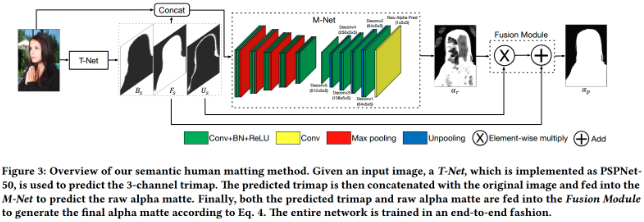M-Net 接受待抠图像(前景与背景的 RGB 3通道合成)以及语义分割模型输出的3通道预测(Fs，Bs，Us) 拼接而成的 6 通道输入，经过编码器提取图像特征之后，由解码器得到预测Alpha($\alpha _r$). 如果语义分割模型分割的精度较高，那么可以认为 Fs 和 Bs 对应的区域已经很好的抠出了大部分的前景和背景，唯一需要提升准确率的是待抠对象的边缘区域，所以模型的第二阶段 M-Net 的目的就是细化的预测边缘区域(这正是 Deep Image Matting 要干的事情)，两部分结合即得到最终的预测：

$$F_s + U_s \alpha _r$$

$$预测的前景 = 确定区域上的前景 + 未知区域上的前景$$

$$P(前景) = P(确定区域上前景) + P(未知区域上前景)$$

$$\alpha = P(F) = P(F|known) P(known) + P(F|unknown) P(unknown)$$

$$\alpha = \frac{F_s}{F_s + B_s}(F_s + B_s) + \alpha _r U_s = F_s + U_s \alpha _r$$## 1. DIM 模型实现

Deep Image Matting 原文的模型如下：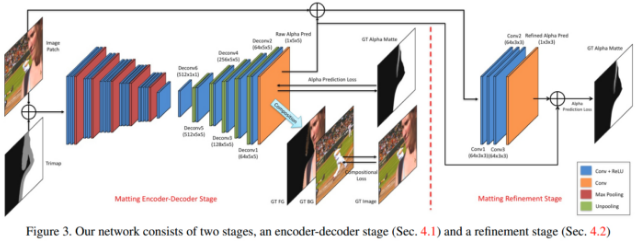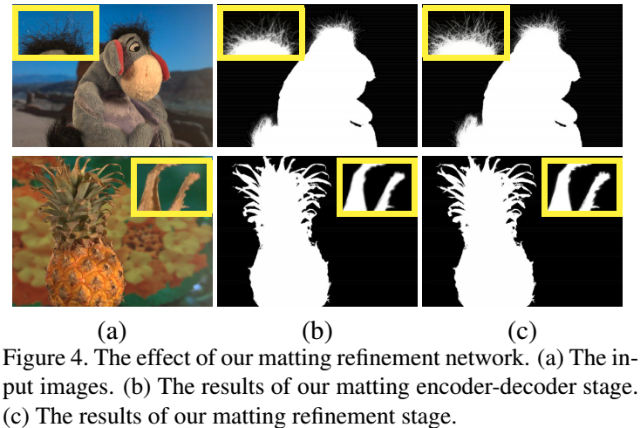## 2. DIM 损失函数

 - 编码器阶段预测的 alpha 通道和真实的 alpha 通道的损失；

 - 编码器阶段使用预测的 alpha 合成的图像和真实的 alpha 合成的图像的损失；

 - 细化阶段预测的 alpha 通道和真实的 alpha 通道的损失.

 - 预测与真实 alpha 通道之间的损失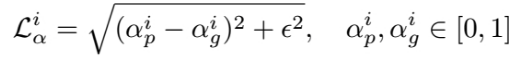- 由前景、背景和 alpha 通道合成图像之间的损失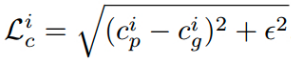## 3. SHM M-Net

Deep Image Matting 虽然论文上报告的效果很惊人，但实际实现时(在个人应用数据集上)泛化性能不够理想.

Semantic Human Matting(SHM)这篇论文的 M-Net 在以上基础上做了一些简化和修改.

## 4. Python 模型定义

# -*- coding: utf-8 -*-
"""
Created on Sun Jul 21 07:08:58 2019

@author: shirhe-lyh

Implementation of paper:
Deep Image Matting, Ning Xu, eta., arxiv:1703.03872
"""

import torch
import torchvision as tv

VGG16_BN_MODEL_URL = 'https://download.pytorch.org/models/vgg16_bn-6c64b313.pth'

VGG16_BN_CONFIGS = {
'13conv':
[64, 64, 'M', 128, 128, 'M', 256, 256, 256, 'M', 512, 512, 512,
'M', 512, 512, 512],
'10conv':
[64, 64, 'M', 128, 128, 'M', 256, 256, 256, 'M', 512, 512, 512]
}

def make_layers(cfg, batch_norm=False):
"""
Copy from: torchvision/models/vgg.

Changs retrue_indices in MaxPool2d from False to True.
"""
layers = []
in_channels = 3
for v in cfg:
if v == 'M':
layers += [torch.nn.MaxPool2d(kernel_size=2, stride=2,
return_indices=True)]
else:
conv2d = torch.nn.Conv2d(in_channels, v, kernel_size=3, padding=1)
if batch_norm:
layers += [conv2d, torch.nn.BatchNorm2d(v),
torch.nn.ReLU(inplace=True)]
else:
layers += [conv2d, torch.nn.ReLU(inplace=True)]
in_channels = v
return torch.nn.Sequential(*layers)

class VGGFeatureExtractor(torch.nn.Module):
"""Feature extractor by VGG network."""

def __init__(self, config=None, batch_norm=True):
"""
Constructor.

Args:
config: The convolutional architecture of VGG network.
batch_norm: A boolean indicating whether the architecture
include Batch Normalization layers or not.
"""
super(VGGFeatureExtractor, self).__init__()
self._config = config
if self._config is None:
self._config = VGG16_BN_CONFIGS.get('10conv')
self.features = make_layers(self._config, batch_norm=batch_norm)
self._indices = None

def forward(self, x):
self._indices = []
self._pre_pool_shapes = []
for layer in self.features:
if isinstance(layer, torch.nn.modules.pooling.MaxPool2d):
self._pre_pool_shapes.append(x.size())
x, indices = layer(x)
self._indices.append(indices)
else:
x = layer(x)
return x

def vgg16_bn_feature_extractor(config=None, pretrained=True, progress=True):
model = VGGFeatureExtractor(config, batch_norm=True)
if pretrained:
state_dict = tv.models.utils.load_state_dict_from_url(
VGG16_BN_MODEL_URL, progress=progress)
model.load_state_dict(state_dict, strict=False)
return model

class DIM(torch.nn.Module):
"""Deep Image Matting."""

def __init__(self, feature_extractor):
"""
Constructor.

Args:
feature_extractor: Feature extractor, such as VGGFeatureExtractor.
"""
super(DIM, self).__init__()
# Head convolution layer, number of channels: 4 -> 3
self._head_conv = torch.nn.Conv2d(in_channels=4, out_channels=3,
kernel_size=5, padding=2)
# Encoder
self._feature_extractor = feature_extractor
self._feature_extract_config = self._feature_extractor._config
# Decoder
self._decode_layers = self.decode_layers()
# Prediction
self._final_conv = torch.nn.Conv2d(self._feature_extract_config, 1,
kernel_size=5, padding=2)
self._sigmoid = torch.nn.Sigmoid()

def forward(self, x):
x = self._head_conv(x)
x = self._feature_extractor(x)
indices = self._feature_extractor._indices[::-1]
unpool_shapes = self._feature_extractor._pre_pool_shapes[::-1]
index = 0
for layer in self._decode_layers:
if isinstance(layer, torch.nn.modules.pooling.MaxUnpool2d):
x = layer(x, indices[index], output_size=unpool_shapes[index])
index += 1
else:
x = layer(x)
x = self._final_conv(x)
x = self._sigmoid(x)
return x

def decode_layers(self):
layers = []
strides = 
channels = []
config_reversed = self._feature_extract_config[::-1]
for i, v in enumerate(config_reversed):
if v == 'M':
strides.append(2)
channels.append(config_reversed[i+1])
channels.append(channels[-1])
in_channels = self._feature_extract_config[-1]
for stride, out_channels in zip(strides, channels):
if stride == 2:
layers += [torch.nn.MaxUnpool2d(kernel_size=2, stride=2)]
layers += [torch.nn.Conv2d(in_channels, out_channels,
kernel_size=5, padding=2),
torch.nn.BatchNorm2d(num_features=out_channels),
torch.nn.ReLU(inplace=True)]
in_channels = out_channels
return torch.nn.Sequential(*layers)

def loss(alphas_pred, alphas_gt, images=None, epsilon=1e-12):
losses = torch.sqrt(
torch.mul(alphas_pred - alphas_gt, alphas_pred - alphas_gt) +
epsilon)
loss = torch.mean(losses)
if images is not None:
images_fg_gt = torch.mul(images, alphas_gt)
images_fg_pred = torch.mul(images, alphas_pred)
images_fg_error = images_fg_pred - images_fg_gt
losses_image = torch.sqrt(
torch.mul(images_fg_error, images_fg_error) + epsilon)
loss += torch.mean(losses_image)
return loss

#
def loss2(alphas_pred, alphas_gt, masks, images=None, epsilon=1e-12):
diff = alphas_pred - alphas_gt
diff = diff * masks
num_unkowns = torch.sum(masks) + epsilon
losses = torch.sqrt(torch.mul(diff, diff) + epsilon)
loss = torch.sum(losses) / num_unkowns
if images is not None:
images_fg_gt = torch.mul(images, alphas_gt)
images_fg_pred = torch.mul(images, alphas_pred)
images_fg_diff = images_fg_pred - images_fg_gt
images_fg_diff = images_fg_diff * masks
losses_image = torch.sqrt(
torch.mul(images_fg_diff, images_fg_diff) + epsilon)
loss += torch.sum(losses_image) / num_unkowns
return loss

Pytorch 的官方是带有 VGG 系列模型的，使用也很方便，比如使用带批标准化层的 VGG16 直接写为：

vgg = torchvision.models.vgg16_bn(pretrained=True)

layers += [nn.MaxPool2d(kernel_size=2, stride=2)]

layers += [torch.nn.MaxPool2d(kernel_size=2, stride=2, return_indices=True)]

def forward(self, x):
self._indices = []
for layer in self.features:
if isinstance(layer, torch.nn.modules.pooling.MaxPool2d):
x, indices = layer(x)
self._indices.append(indices)
else:
x = layer(x)
return x

unpool = torch.nn.MaxUnpool2d(kernel_size=2, stride=2)
conv = torch.nn.Conv2d(in_channels, out_channels, kernel_size=5, padding=2)

x = unpool(x, indices)
x = conv(x)

DIM 类的 decode_layers 函数就是整个的解码器的定义. 它看起来有点晦涩，其实不难理解. 我们看编码器阶段的配置：

[64, 64, 'M', 128, 128, 'M', 256, 256, 256, 'M', 512, 512, 512, 'M', 512, 512, 512]

[512, 'U', 512, 'U', 256, 'U', 128, 'U', 64]

## 5. 训练过程### 5.1. 数据准备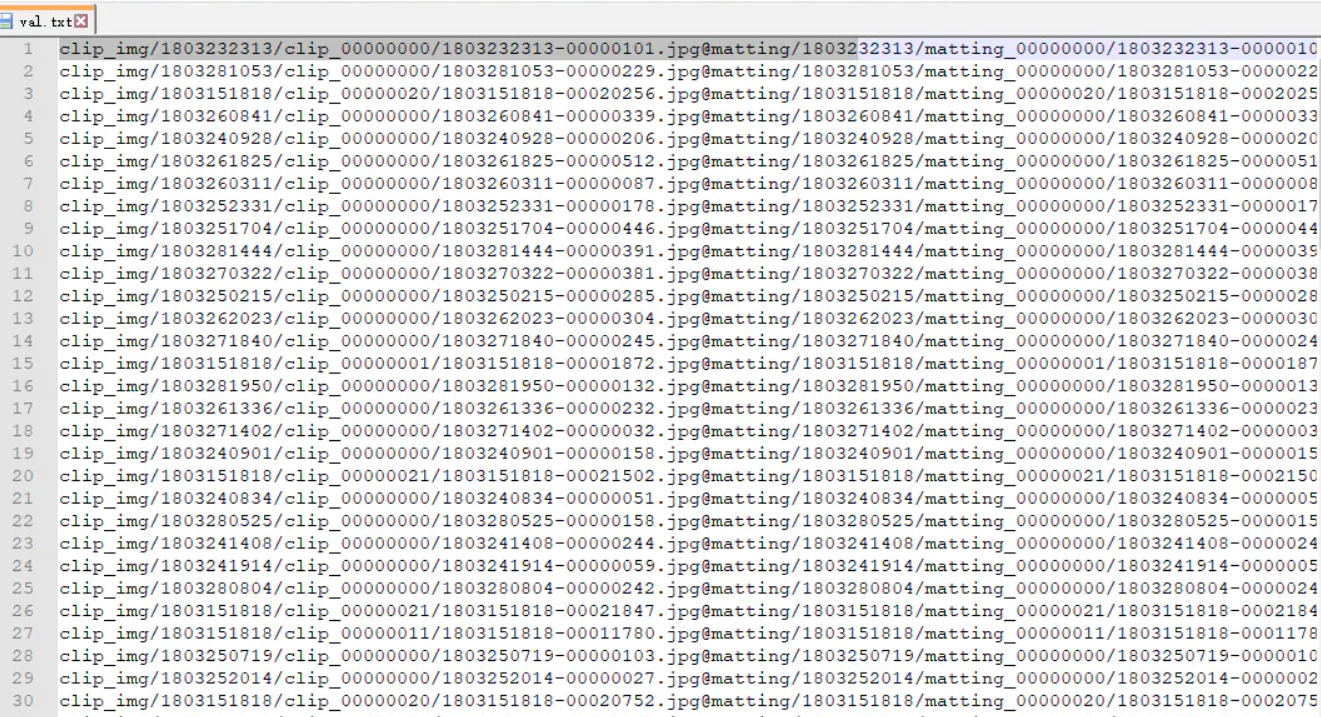train.txt 和 val.txt 分别记录了训练和验证图像的路径，每一行对应一张图像的 4 个路径，分别是原图像路径(3 通道)、透明图路径(4 通道)、alpha 通道图像路径、mask 路径，它们通过 @ 符号分隔.

### 5.2. 训练

python3 train.py --root_dir "xxx/Matting_Human_Half" [--gpu_indices 0 1 ...]

--gpu_indices 0

--gpu_indices 1 3

tensorboard --logdir ./models/logs

python3 predict.py

### 5.3. 其它数据集上训练

[[image_path, alpha_path],
[image_path, alpha_path],
...
[image_path, alpha_path]]

crop_sizes = [320, 480, 600, 800]

## 6. DIM 数据集上的复现

### 6.1. 数据准备

bg_image_root_dir 填写背景图像的文件夹路径，

output_dir 填写合成图像的保持文件夹路径.

num_bg_images_per_fg 表示一张前景图像对应多少张背景图像，论文里这个值取 100(这里为了减少训练时间，本文取的是 50，读者根据具体情况修改).

train.txt 文件里每一行对应 4 个路径，分别是合成图像路径、前景图像路径、alpha 通道路径、背景图像路径，val.txt 里除了缺少合成图像路径之外，其它顺序一致.

crop_sizes = [320, 480, 640]

### 6.2. 训练过程

python3 train.py --annotation_path "./data_dim/train.txt" [gpu_indices 0 1 2 ...]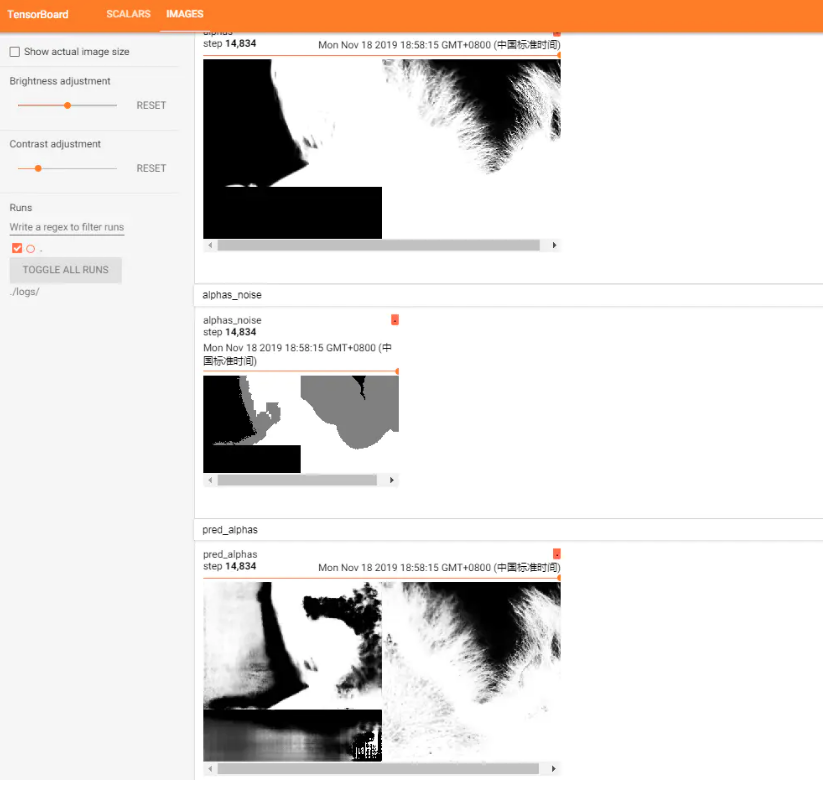### 6.3. 结果展示

python3 predict_trimap.py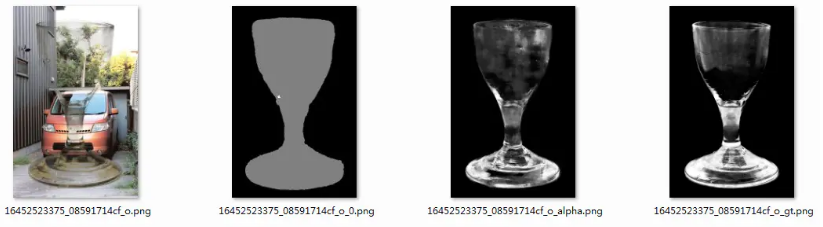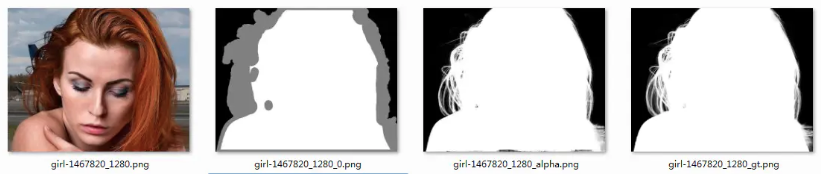Last modification：November 27th, 2020 at 05:09 pm